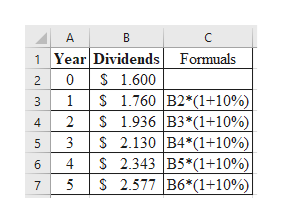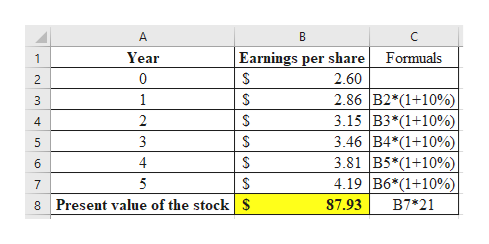# Suppose that a firm’s recent earnings per share and dividend per share are \$2.60 and \$1.60, respectively. Both are expected to grow at 10 percent. However, the firm’s current P/E ratio of 25 seems high for this growth rate. The P/E ratio is expected to fall to 21 within five years. Compute the dividends over the next five years. (Do not round intermediate calculations. Round your answers to 3 decimal places.)Compute the value of this stock in five years. (Do not round intermediate calculations. Round your answer to 2 decimal places.)Calculate the present value of these cash flows using a 12 percent discount rate. (Do not round intermediate calculations. Round your answer to 2 decimal places.)

Question
6 views

Suppose that a firm’s recent earnings per share and dividend per share are \$2.60 and \$1.60, respectively. Both are expected to grow at 10 percent. However, the firm’s current P/E ratio of 25 seems high for this growth rate. The P/E ratio is expected to fall to 21 within five years.

Compute the dividends over the next five years. (Do not round intermediate calculations. Round your answers to 3 decimal places.)

Compute the value of this stock in five years. (Do not round intermediate calculations. Round your answer to 2 decimal places.)

Calculate the present value of these cash flows using a 12 percent discount rate. (Do not round intermediate calculations. Round your answer to 2 decimal places.)

check_circle

Step 1

Calculate the dividend over the next five years as follows:help_outlineImage TranscriptioncloseA B 1 Year DividendsFormuals 0S 1.600 2 S 1.760 B2*(1+10% S 1.936 B3*(1+10%) S 2.130 B4*(1+10%) S 2.343 B5*(1+10%) S 2.577 B6*(1+10%) 1 3 4. 3 5 4 6 5 7 LO fullscreen
Step 2

Calculate the present value of...help_outlineImage TranscriptioncloseВ C Earnings per share Year Formuals 0 2.60 2 2.86 B2*(1+10% 3.15 B3 (1+10%) 3.46 B4*(1+10% 3.81 B5*(1+10% 4.19 B6*(1+10% 1 3 2 3 5 4 6 5 7 Present value of the stock 87.93 B7*21 8 st LO LO fullscreen

### Want to see the full answer?

See Solution

#### Want to see this answer and more?

Solutions are written by subject experts who are available 24/7. Questions are typically answered within 1 hour.*

See Solution
*Response times may vary by subject and question.
Tagged in